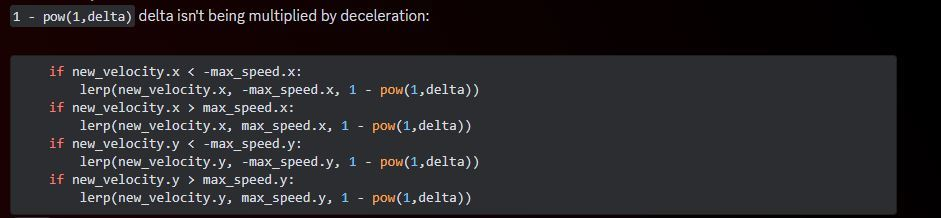g

# How would one make the character decelerate over time when coming out of sprint?

gbtrob

I noticed that even in Nathan's version, when you release the sprint key, your speed is instantly clamped to (+/-)500. In my case, it bugs me, because I purposefully wanted to add a Sprint state, thinking it could be good for a more complex character controller. The result is that my character can't carry momentum into a jump, because switching to the Air state immediately clamps it.

If I wanted to make it so any time the player is somehow above the max speed, they decelerate to the max speed over time, how could I do it properly? I tried lerp() but it still keeps on accelerating faster than it decelerates.

`static func calculate_velocity(		old_velocity: Vector2,		max_speed: Vector2, # limite (clamp)		acceleration: Vector2,		deceleration: Vector2,		delta: float,		move_direction: Vector2	) -> Vector2:	var new_velocity: = old_velocity	new_velocity.y += move_direction.y * acceleration.y * delta	if move_direction.x:		new_velocity.x += move_direction.x * acceleration.x * delta	elif abs(new_velocity.x) > 0.1:		new_velocity.x -= deceleration.x * delta * sign(new_velocity.x)		new_velocity.x = new_velocity.x if sign(new_velocity.x) == sign(old_velocity.x) else 0	#new_velocity.x = clamp(new_velocity.x, -max_speed.x, max_speed.x)	#new_velocity.y = clamp(new_velocity.y, -max_speed.y, max_speed.y)	if new_velocity.x < -max_speed.x:		lerp(new_velocity.x, -max_speed.x, 1 - pow(1,delta))	if new_velocity.x > max_speed.x:		lerp(new_velocity.x, max_speed.x, 1 - pow(1,delta))	if new_velocity.y < -max_speed.y:		lerp(new_velocity.y, -max_speed.y, 1 - pow(1,delta))	if new_velocity.y > max_speed.y:		lerp(new_velocity.y, max_speed.y, 1 - pow(1,delta))	return new_velocity`
• a
andrewjhaugen replied

One of my colleagues looked at this and pointed this out.